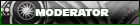2nd Generation Specific 1986-1992 Discussion

# Technical Turbo Question.

Senior Member

Join Date: Feb 2003
Location: Miami, Florida
Posts: 1,591OK i just learned how to read a compressor efficiency map. I also learned how to calculate required flow rate and psi to obtain projected HP but my calculations seem kinda weird.

First Flow Rate

WA= hp*a/f*BSFC/60

Where:

· Wa = Airflowactual (lb/min)

· HP = Horsepower Target (flywheel)

· a/f= Air/Fuel Ratio

· BSFC/60= Brake Specific Fuel Consumption ( ) ÷ 60 (to convert from hours to minutes)

My Calculation based on my 450 flywheel HP target

450*12.0*.60/60 = 54 LB/min (WA)

Now PSI

MAP = WA*R*(460+100)

---------------------

VE*n/2*VD

where:

· MAPreq = Manifold Absolute Pressure (psia) required to meet the horsepower target

· Wa = Airflowactual(lb/min)

· R = Gas Constant = 639.6

· Tm = Intake Manifold Temperature (degrees F)

· VE = Volumetric Efficiency

· N = Engine speed (RPM)

· Vd = engine displacement (Cubic Inches, convert from liters to CI by multiplying by 61.02, ex. 2.0 liters * 61.02 = 122 CI)

My calculations

54*639.6*(460+100) = 19341280 = 78 PSIA - 14.7 Atmospheric = 63.3 PSI here is the problem.

------------------------ ------------

.90*7000/2*79 = 248850

if engine displacement is calculated 1.3*61.02 that is 79 but if i calculate it as a 2.6 = 159 ci which is both rotors it is a more resonable result

54*639.6*(460+100) = 19341280 = 39PSIA - 14.7 Atmospheric = 24.3 PSI see

------------------------ ------------

.90*7000/2*159 = 500850

24psi is more along the lines but why did i get 63 psi on my first calculation.

and dosent 24 psi sound to high for 450 hp?Super ModeratorJoin Date: Sep 2002
Location: Savannah, Ga
Posts: 2,763That equation was for 4 stroke engines, which require 2 revolutions to complete a complete cycle. Rotaries only require one revolution, so you need to use the 2 stroke equation for airflow requirements on a rotary. Your 24 psi answer was correct in this case.Senior Member

Join Date: May 2002
Location: California
Posts: 22,466Originally Posted by mazdaspeed7' post='851424' date='Jan 3 2007, 01:24 PM

That equation was for 4 stroke engines, which require 2 revolutions to complete a complete cycle. Rotaries only require one revolution, so you need to use the 2 stroke equation for airflow requirements on a rotary. Your 24 psi answer was correct in this case.

or you double the displacement, 2613cc instead of 1308.

also .6 bsfc is a little low, .7 is more realistic. well in reality, its going to end up somewhere in the middle. i like to calculate with both, and that gives you a range.

also you might wanna do the calcs at half the rpms, 3500, 4000 that will give you some idea when you'll see full boost.Senior Member

Join Date: Feb 2003
Location: Miami, Florida
Posts: 1,591How would I go about calculating for a 2 stroke air flow requirementsSenior Member

Join Date: May 2002
Location: California
Posts: 22,466Originally Posted by RX7 13B 4 UR AZZ' post='851608' date='Jan 4 2007, 03:06 PM

How would I go about calculating for a 2 stroke air flow requirements

use double the displacement or half the rpms.

basically a 4 stroke piston engine fires its RATED displacement (all cylinders) in 2 revolutions of the crankshaft, or 720 degrees.

a 2 stroke engine, will fire all the cylinders in 360 degrees.

a rotary, is a 4 stroke, but fires 1308cc in one revolution, 2616 in two.

since the formula is for a 4 stroke piston engine, to make apples apples, you need to plug in the value that the rotary would have after 720 degrees of crank rotation, which is double the 1.3, or 2.6, 2616cc or 160CID....

aight?Senior Member

Join Date: Feb 2003
Location: Miami, Florida
Posts: 1,59154*639.6*(460+100) = 19341280 = 39PSIA - 14.7 Atmospheric = 24.3 PSI see

------------------------ ------------

.90*7000/2*159 = 500850

^

^

^

I did that here and i still got 24.3 psi for 450 hpSuper ModeratorJoin Date: Sep 2002
Location: Savannah, Ga
Posts: 2,763Originally Posted by RX7 13B 4 UR AZZ' post='852166' date='Jan 7 2007, 08:19 PM

54*639.6*(460+100) = 19341280 = 39PSIA - 14.7 Atmospheric = 24.3 PSI see

------------------------ ------------

.90*7000/2*159 = 500850

^

^

^

I did that here and i still got 24.3 psi for 450 hp

What turbo are you using?

Regardless, thats still a very basic calculation, while engines are very complex. You will likely find that it doesnt correspond all that well to reality. It will give you a ballpark figure, but dont expect any more than that from the numbers.Forum
Replies
Last Post
elnr_uk
3rd Generation Specific
1
01-16-2006 03:52 PM
94touring
Insert BS here
13
03-02-2005 08:40 AM
SoRRoW
Insert BS here
2
09-29-2002 03:10 AM
sleeperRX7
Insert BS here
2
05-03-2002 10:26 AM

Currently Active Users Viewing This Thread: 1 (0 members and 1 guests)Show Printable VersionEmail this Page